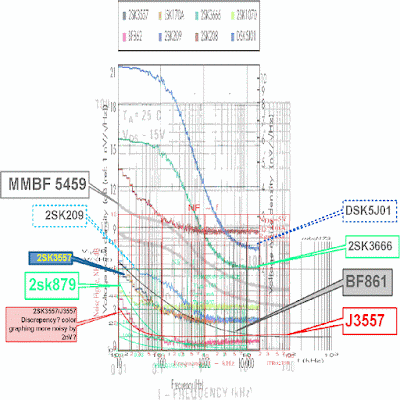### :: JFET low noise amplifier exploration

This post is inspired by GK's thread --> http://www.eevblog.com/forum/projects/low-noise-amplifier/?all, GK made a very impressive low noise amplifier using 12 paralleled JFETs. Preceding his post are also countless other very good reads :

[color=red]- http://www.eevblog.com/forum/metrology/characterising-low-frequency-noise/?all <--- best thread imo (by alanambrose) :popcorn:[/color] so much info
- http://www.eevblog.com/forum/projects/low-frequency-very-low-level-dc-biased-noise-measurements/ <-- (by janaf) <-- blackdog's ULNA version inside
- http://www.eevblog.com/forum/metrology/diy-low-frenquency-noise-meter/?all <--- ULNA by zlymex inside
- http://www.rroij.com/open-access/smallsignal-amplifier-with-jfets-in-tripledarlington-topology.php <--- understanding of JFET amplifier
needless to say, many related videos lurk in youtube, etc.this chart is combined overlay of various JFETs with the scale approximately normalized.

of interest is the BF862 (NXP), a newer release 2SK3557 (ONSEMI) and possibly 2SK209. (in the graph there are 2 BF862 plots, 1 assumed to be of 1kHz and the other 100kHz from the std PDF)

BF862 spice model (NXP) SOT23
ver 1
http://www.diyaudio.com/forums/solid-state/281387-bf862-ltspice-model.html
https://e2e.ti.com/support/development_tools/webench_design_center/f/234/t/236449
we shall call this the L1 spice model

.model BF862L NJF(Beta=.0478 Betatce=-.5 Rd=.8 Rs=7.5 Lambda=.0373 Vto=-.57093 Vtotc=-.002 Is=424.60E-12 Isr=2.995p N=1 Nr=2 Xti=3 Alpha=-.001 Vk=59.97 Cgd=7.4002E-12 M=.6015 Pb=.5 Fc=.5 Cgs=8.2890E-12 Kf=87.5E-18 Af=1.2)

the SPICE model is modified to allow for changes in Idss

.model BF862-2mA NJF(beta= 0.026594 VTO= -0.2964 lambda= 0.036629 Rs= 0.4684 Is= 9.36E-14 N= 1.245 Betatce=-0.5 Vtotc=-0.002 Isr=2.995p Nr=2 Xti=3 Alpha=-0.001 Vk=59.97 Cgd=7.4002E-12 Pb=0.5 Fc=0.5 Cgs=8.2890E-12 Kf=87.5E-18 Af=1.2)

.model BF862-5mA NJF(beta= 0.031925 VTO= -0.3648 lambda= 0.036629 Rs= 2.01 Is= 9.36E-14 N= 1.245 Betatce=-0.5 Vtotc=-0.002 Isr=2.995p Nr=2 Xti=3 Alpha=-0.001 Vk=59.97 Cgd=7.4002E-12 Pb=0.5 Fc=0.5 Cgs=8.2890E-12 Kf=87.5E-18 Af=1.2)

.model BF862-8mA NJF(beta= 0.037256 VTO= -0.4332 lambda= 0.036629 Rs= 3.5506 Is= 9.36E-14 N= 1.245 Betatce=-0.5 Vtotc=-0.002 Isr=2.995p Nr=2 Xti=3 Alpha=-0.001 Vk=59.97 Cgd=7.4002E-12 Pb=0.5 Fc=0.5 Cgs=8.2890E-12 Kf=87.5E-18 Af=1.2)

.model BF862-11mA NJF(beta= 0.042587 VTO= -0.5016 lambda= 0.036629 Rs= 5.0917 Is= 9.36E-14 N= 1.245 Betatce=-0.5 Vtotc=-0.002 Isr=2.995p Nr=2 Xti=3 Alpha=-0.001 Vk=59.97 Cgd=7.4002E-12 Pb=0.5 Fc=0.5 Cgs=8.2890E-12 Kf=87.5E-18 Af=1.2)

.model BF862-15mA NJF(beta= 0.049998 VTO= -0.5967 lambda= 0.036629 Rs= 7.234 Is= 9.36E-14 N= 1.245 Betatce=-0.5 Vtotc=-0.002 Isr=2.995p Nr=2 Xti=3 Alpha=-0.001 Vk=59.97 Cgd=7.4002E-12 Pb=0.5 Fc=0.5 Cgs=8.2890E-12 Kf=87.5E-18 Af=1.2)

.model BF862-25mA NJF(beta= 0.067465 VTO= -0.8208 lambda= 0.036629 Rs= 12.2835 Is= 9.36E-14 N= 1.245 Betatce=-0.5 Vtotc=-0.002 Isr=2.995p Nr=2 Xti=3 Alpha=-0.001 Vk=59.97 Cgd=7.4002E-12 Pb=0.5 Fc=0.5 Cgs=8.2890E-12 Kf=87.5E-18 Af=1.2)

in both, the Af is =1.2 to emulate a more realistic noise character near 1Hz. both models perform very very differently in simulators.

What are in JFET SPICE parameters?

.model BF862
NJF
(
BETA=47.800E-3 [Transconductance parameter A/VV]
B [doping tail extending parameter 1/V]
Betatce=-.5 [Transconductance param exp temp coefficient A/VV -PSPICE =0.0001]
RD=.8 [Drain ohmic resistance Ω]
RS=7.5000 [Source ohmic resistance Ω]
LAMBDA=37.3E-3 [channel length modulation parameter 1/V]
VTO=-0.57093 [Zero-bias threshold voltage V]
Vtotc=-2.0000E-3 [Threshold voltage temperature coefficient V/˚C -PSPICE=0]
IS=424.60E-12 [Parasitic diode saturation current A]
Isr=2.995p [Gate junction recombination current parameter A -PSPICE=0]
N=1 [emission coefficient -PSPICE=1]
Nr=2 [Emission coefficient for Isr -PSPICE=2]
Xti=3 [saturation-current temp -PSPICE=3]
Alpha=-1.0000E-3 [Ionization coefficient 1/V -PSPICE=0]
Vk=59.97 [Ionization knee voltage V -PSPICE=0]
CGD=7.4002E-12 [gate to drain capacitance F]
M=.6015 [grading coefficient -PSPICE=0.5]
PB=.5 [Gate junction potential V]
FC=.5 [Coefficient for forward-depletion capacitance]
CGS=8.2890E-12 [gate to source capacitance F]
KF=87.5E-18 [Flicker noise coefficient ]
AF=1 [Flicker noise exponent]
TNOM [parameter temperature 27˚C]
)

2SK3557/NSVJ3557 spice model (ONSEMI) SOT23/SC-59

.MODEL 2SK3557 NJF(BETA=0.026 VTO=-0.80 LAMBDA=0.038 RD=5.6 RS=6.5 IS=0.558e-15 CGS=7.62e-12 CGD=7.62e-12 M=0.33 PB=0.6 FC=0.5 N=1.016 )

2SK209 spice model (toshiba) SOT23/SC-59
http://www.diyaudio.com/forums/software-tools/62144-2sk209-2sj106-spice-models.html

.MODEL 2SK209 NJF(BETA=0.021 VTO=-0.7869 LAMBDA=0.00615 RD=12.19 RS=16.75 IS=2.61e-15 CGS=14.78e-12 CGD=2.613e-15 PB=0.3754 FC=0.5 N=1 )

from datasheet we understand BF862 to have noise density of 0.8nV/Hz^0.5 (@ 100kHz)

Someone else has the noise data @1kHz
BF862 ~ 1.1nV/Hz^0.5
2SK3557 ~ 1.3nV/Hz^0.5
2SK209 ~ 1.3nV/Hz^0.5

if we then wish to know the approx voltage noise from 0.01Hz ~ 10Hz (BW = 9.99Hz, thermal noise of 1kohm resistor is ~ 4.1nV RMS / -167.7 dbV)
BF862 ~ 1.1*(9.99^0.5) = 3.5nV RMS (-169.1 dbV)
2SK3557 ~ 1.3*(9.99^0.5) = 4.1nV RMS (-167.7 dbV)

so specification wise, it appears the BF862 will always have the upper hand in low noise.
in simulation, to resolve very low voltages/frequencies, certain special simulation options need to be changed ~ RELTOL = 1e-11, TRTOL = 5e-06, VNTOL = 1e-15, CHGTOL = 1e-15, ABSTOL = 1e-15, METHOD = GEAR, TNOM = 35.
after mucking around with the simulations.

It seems highly likely to couple 4 stages of gains and produce a +100dBV amplifier in various flavors of gain each stage.